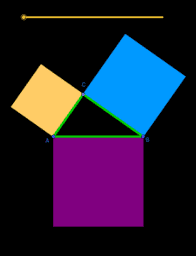# Proofs of the Pythagorean Theorem

This book contains a few visual proofs of the Pythagorean Theorem. I'll continue adding to it. As you play with each applet, try to (1) describe what's going, and (2) explain what conclusions we can draw from it. If you have a hard time with one of these pages, please let me know so that I can improve it. If you want to know more about shear transformations, try https://www.geogebra.org/m/yZQNWxs6.
•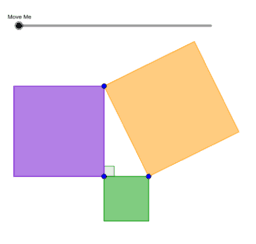### Pythagorean Theorem via Shear Transformation

•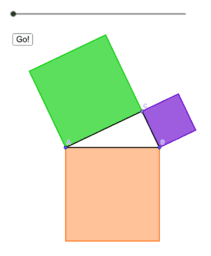### Second Proof of Pythagorean Theorem by Shear Transformation

•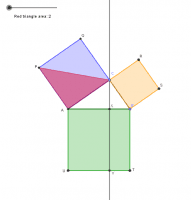### Euclid's Proof

•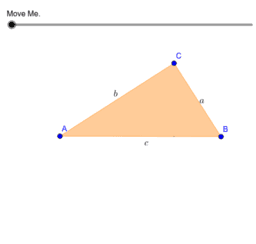### Pythagorean Theorem via Similar Triangles

•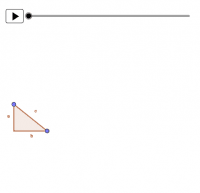### Pythagorean Theorem by Scaling

•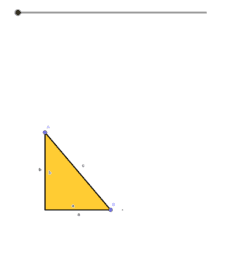### Pythagorean Theorem by Scooting

•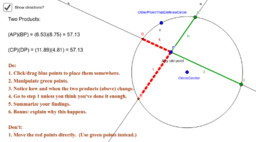### Power Of A Point

•